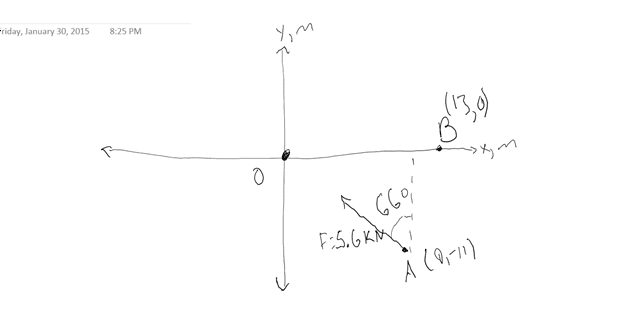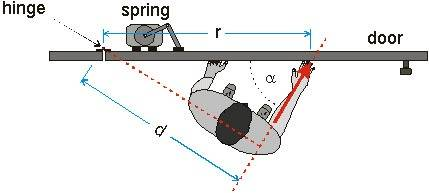# Statics Homework Help

June W.

## Homework Statement

This problem is from WileyPLUS. I've been looking through my book, but none of the methods they present work for me. I guess I just don't know how to use them properly.

Determine the moments of the 5.6-kN force about the point O and about point B.## Homework Equations

I know I can split F into its X and Y components by using Fsin(theta) and Fcos(theta).

## The Attempt at a Solution

I think if I multiply the X and Y components of F by the corresponding coordinate distances between point A and B or A and O and add what I get, I should get the moment but for some reason this isn't giving me the right answer:

9sin(24) + (-11)cos(24) = -6.388
-6.388 * -5.6 = 35.77

and

-4sin(24) + (-11)cos(24) = -11.676
-11.676 * -5.6 = 65.39

All the website tells me is that these answers are wrong. Any help is greatly appreciated! Thanks!

barefeet
Your distance is wrong. Look up the definition of a moment. How is the distance defined? From where to where? Because it isn't always from the point of where your force is applied to thew point you are rotating about

June W.
Isn't the distance for a moment arm the distance between corresponding coordinates? So if the vector tail were at (2,4) and moment were being measured with respect to the origin, wouldn't the distances be 2 units of distance in the X direction and 4 units of distance in the Y direction?

I know it has something to do with the perpendicular distance between the two relevant points, but I can't tell how that changes based on which method is being used to find the moment.

barefeet
So if the vector tail were at (2,4) and moment were being measured with respect to the origin, wouldn't the distances be 2 units of distance in the X direction and 4 units of distance in the Y direction?

That is just the distance between the points, but it is not the distance you are looking for as it is defined for the moment.
Can you quote the definition of moment?
Btw, you don't even have to split the force, you just need to figure out the distance

June W.
The definition in my book (as best I can make it) is that "moment" is really the same as "torque," so the moment is a vector perpendicular to the plane of the body in question.

So I don't need to split the force into X and Y components? How does that work? I know it's possible to calculate the moment using a cross product but I don't know how to do in this case.

Back to the previous example I used, would the distance I need be (2^2 + 4^2)^(1/2) = 4.47?

Would I just multiply this distance by the magnitude of the force vector?

barefeetIf you only need to calculate the magnitude of the moment, then you can just multpily the force with the (correct) distance.

Back to the previous example I used, would the distance I need be (2^2 + 4^2)^(1/2) = 4.47?
No, you need the perpendicular distance, d, NOT r, between the line of action of the force and the point you are rotating about. See the picture for example.

June W.
Am I supposed to use r and theta to find d? I'm still confused.

barefeet
No you have to find out what the distance is from point you are rotating about for example and the line of action of the force. For example if your force goes through the origin then the distance between the origin and the line of action of the force is 0, because as it goes through it the distance between them is 0.
Take a look again at the red arrow in the picture. That is the force. You have to extend it, that is the dashed red line and figure out the shortest distance to your point you are rotating about. That is the other dashed red line,d.

June W.
Is the line of action extended along the angle they provided (66 degrees into the second quadrant from the y axis)?

What do I use to find the shortest distance? I know it intersects the dashed red line at a 90 degree angle, but I don't know what to do with that information.

barefeet
Is the line of action extended along the angle they provided (66 degrees into the second quadrant from the y axis)?

Yes, otherwise it wouldn't be a straight line anymore.

What do I use to find the shortest distance? I know it intersects the dashed red line at a 90 degree angle, but I don't know what to do with that information.

You know that it is perpendicular to your line of action and it goes through the origin. Two pieces of information that should be enough to know what that line is. The intersection of that line with your line of action is the point you are looking for. And the distance from that point to the origin is your distance. The same can be done for point B

June W.
Sorry, I'm still not getting it. Do I need to find the slope of the line of action? I don't know how to find where d intersects the line. I know all three angles in the triangle formed by a line perpendicular to the line of action, but I don't know the lengths of any of the sides. Is there a ratio I'm supposed to find? The length d is the shortest perpendicular distance to intersect the line of action but I'm not sure how that helps me.

barefeet
Yes you need the slope of both lines. You have your line of action which has the form $y = ax + b$ and the line of the shortest distance has the same form. a is the slope. If you have that you can find their intersection by equating them

June W.
Thank you so much! That make so much more sense! I couldn't figure out what I was missing in class but that was probably it. Thanks!